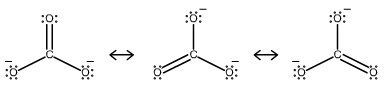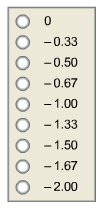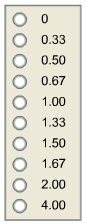Chemistry Practice Problems Bond Order Practice Problems Solution: Consider the resonance structures for the carbonat...

🤓 Based on our data, we think this question is relevant for Professor Wallace's class at LEHMAN-CUNY.

# Solution: Consider the resonance structures for the carbonate ion. How much negative charge is on each oxygen of the carbonate ion? What is the bond order of each carbon-oxygen bond in the carbonate ion?

###### Problem

Consider the resonance structures for the carbonate ion.How much negative charge is on each oxygen of the carbonate ion?What is the bond order of each carbon-oxygen bond in the carbonate ion?###### Solution

Analyze each structure and determine the charges in each atom. The sum of the charges will be the net charge of the molecule

For the bond order, identify the bonds in the structure.

Step 1. Analyze the resonance structures

• Since the charges of the atoms are already given, we can notice that two of O atoms have a charge of -1 where C and O are neutral.View Complete Written Solution

Bond Order

Bond Order

#### Q. Determine the bond order from the molecular orbital diagram of Ne2. Does the bond order calculated agree with what you would draw for Lewis structures...

Solved • Tue Aug 27 2019 16:42:31 GMT-0400 (EDT)

Bond Order

#### Q. Determine the bond order from the molecular orbital diagram of N2. Does the bond order calculated agree with what you would draw for Lewis structures ...

Solved • Tue Aug 27 2019 16:39:30 GMT-0400 (EDT)

Bond Order

#### Q. What is the bond order in NO2+?a. 2b. 3c. 1.5d. 4

Solved • Wed Aug 07 2019 18:05:51 GMT-0400 (EDT)

Bond Order

#### Q. Use a molecular orbital diagram to determine the bond order in the O 2+ ion. Write a valence-electron configuration [(s2s)2] for this ion.

Solved • Tue Aug 06 2019 16:39:32 GMT-0400 (EDT)